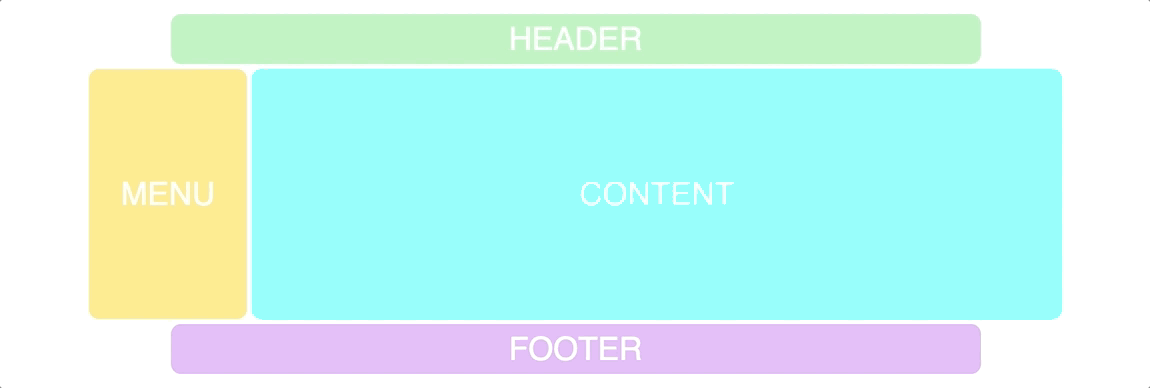## 1. 标记

``````<div class="container">
<div class="content">CONTENT</div>
<div class="footer">FOOTER</div>
</div>``````

## 2. CSS 中的基础设置

``````.container {
display: grid;
grid-template-columns: repeat(12, 1fr);
grid-template-rows: 50px 350px 50px;
grid-gap: 5px;
}``````

## 3. 添加 grid-template-areas

``````.container {
display: grid;
grid-gap: 5px;
grid-template-columns: repeat(12, 1fr);
grid-template-rows: 50px 350px 50px;
grid-template-areas:
"h h h h h h h h h h h h"
"m m c c c c c c c c c c"
"f f f f f f f f f f f f";
}``````

grid-template-areas 属性背后的逻辑是你在代码中创建一个可视化的网格。你可以看到，它有 3 行、12 列。就像我们在定义 grid-template-columns 和 grid-template-rows 一样。

## 4. 把网格区域赋给项目

``````.header {
grid-area: h;
}
grid-area: m;
}
.content {
grid-area: c;
}
.footer {
grid-area: f;
}``````

## 试验布局

``````grid-template-areas:
“h h h h h h h h h h h h”
"c c c c c c c c c c m m”
“f f f f f f f f f f f f”;``````

``````grid-template-areas:
“. h h h h h h h h h h .”
"c c c c c c c c c c m m”
“. f f f f f f f f f f .”;``````

## 添加响应能力

``````@media screen and (max-width: 640px) {
.container {
grid-template-areas:
"m m m m m m h h h h h h"
"c c c c c c c c c c c c"
"f f f f f f f f f f f f";
}
}``````

GIF 链接（总是上传失败，也是醉了）